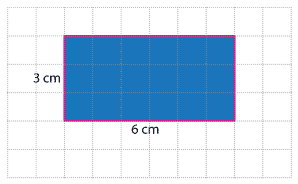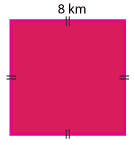### Area of rectangles

The area of a rectangle is the size of the region inside it.

For this rectangle, drawn on centimetre grid paper, the area is 18 square centimetres. We can write square centimetres as cm².We can find the area of a rectangle by multiplying its length by its width.

We know the lengths of the sides of the rectangle above, and can use this to calculate its area. A 6 cm by 3 cm rectangle contains 6 × 3 = 18 squares, each with an area of 1 square centimetre. So the area of the rectangle is 18 square centimetres, or 18 cm².

We usually set this out as follows:

\begin{align}\text{Area}\ &= \ \text{length} \ × \ \text{width} \\ &= \ 6 × 3 \\ &= \ 18 \ \text{cm²}.\end{align}

We call this the formula for calculating the area of rectangles, and write it as:

Area = length × width.

When we are working with squares we can use the properties of a square to calculate its area. Since the side lengths of the square are equal, the formula becomes

Area = length × length.

So for the square on the right:\begin{align}\text{Area}\ &= 8 × 8\\&= 64\ \text{km²}\end{align}